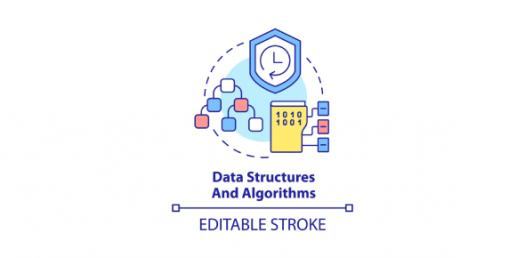# Algorithms, Linear Search, Binary Search

9 Questions | Attempts: 1087
ShareSettings• 1.
The "running time" of an algorithm refers to
• A.

How much time it takes for the program to run

• B.

The number of steps it takes to complete with respect to the size of the input

• C.

How much time it takes to write the program

• 2.
A loop invariant is
• A.

The steps of the algorithm that take place at each iteration of the loop

• B.

The part of the data that doesn't change from one iteration of the loop to the next

• C.

A statement about the data which reflects the progress made at each iteration toward the goal

• 3.
Let's say you do a google search for "pacman". The "key" in this scenario is:
• A.

The top hit (the first web page listed in the search results)

• B.

The place in each web page where the pattern "pacman" is found

• C.

The word "pacman"

• 4.
A "precondition" with respect to algorithms is:
• A.

The initial setup steps made before the main work of the algorithm is done

• B.

A condition that may prevent you from getting insurance

• C.

A true statement that can be made about the data before the algorithm is applied

• 5.
For linear search, the "best case scenario" (when the algorithm completes with the fewest number of steps) is when:
• A.

The first item equals the key

• B.

The middle item equals the key

• C.

The data does not contain the key

• 6.
For linear search, the "worst case scenario" (when the algorithm takes the most number of steps) is when:
• A.

The first item equals the key

• B.

The middle item equals the key

• C.

The data does not contain the key

• 7.
For binary search, the "best case scenario"  (when the algorithm will completes in the fewest number of steps) is when:
• A.

The first item equals the key

• B.

The middle item equals the key

• C.

The data does not contain the key

• 8.
For binary search, the "worst case scenario" (when the algorithm takes the most number of steps) is when:
• A.

The first item equals the key

• B.

The middle item equals the key

• C.

The data does not contain the key

• 9.
Note that 21 = 2, and 22 = 4, and 23 = 8 and so on.In these relationships the term "log base 2" refers to the exponent.For example, "log base 2 of 8" is 3, and "log base 2 of 4 is 2". We can think of "log base 2 of n" as being the number of times you can cut n in half until you get 1. For example, you can cut 8 in half 3 times. In general, a "divide and conquer" algorithm that is always cutting its data in half has a running time of "log base 2 of n". What is "log base 2 of 64"? In other words, how many times can you cut 64 in half?
• A.

6

• B.

5

• C.

7

## Related TopicsBack to top
×

Wait!
Here's an interesting quiz for you.